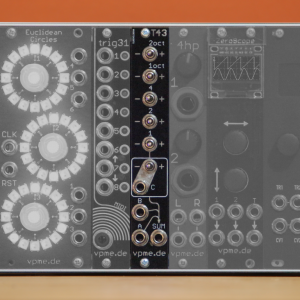T43

OUT NOW: T43 – a switchable precision adder and 43 semitone transposer in 4hpthe SUM output gives the sum of CV inputs A and B and can add or subtract CV input C. the switches allow to add or subtract 1, 2, 4, 12 or 24 semitones so a total of 43 semitones or 3.5 octaves can be added or subtracted from the CV sum.

e.g to add a fourth (5 semitones) use switches +1 and +4, to add a minor seventh (10 semitones) use switches +1 oct and -2

T43 uses precision opamps and voltage references in order to give you perfect tuning across the whole transposition range

T43 on ModularGrid

T43 User Manual